# Addition and subtraction of fractions

### Term 2 starting in week 10 :: Estimated time: 3 weeks

• Understand representations of fractions
• Convert between mixed numbers and fractions
• Add and subtract unit fractions with the same denominator
• Add and subtract fractions with the same denominator
• Add and subtract fractions from integers expressing the answer as a single fraction
• Understand and use equValent fractions
• Add and subtract fractions where denominators share a simple common multiple
• Add and subtract fractions with any denominator
• Add and subtract •mproper fractions and mixed numbers
• Use fractions in algebraic contexts
• Use equivalence to add and subtract decimals and fractions

For higher-attaining pupils:

Add and subtract simple algebraic fractions

This page should remember your ticks from one visit to the next for a period of time. It does this by using Local Storage so the information is saved only on the computer you are working on right now.

## Lesson Starters

Here are some suggestions for whole-class, projectable resources which can be used at the beginnings of each lesson in this block.

### 1st Lesson#### Coins On The Table

A puzzle about the number of coins on a table given information about fractions of them.

### 2nd Lesson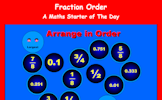#### Fraction Order

Arrange the fractions and decimals in order from smallest to largest.

### 3rd Lesson#### Giraffe

The height of this giraffe is three and a half metres plus half of its height. How tall is the giraffe?

### 4th Lesson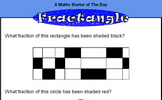#### Fractangle

Can you see what fractions of the shapes have been shaded? It is not as difficult as it first appears.

### 5th Lesson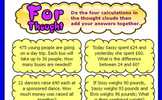### 6th Lesson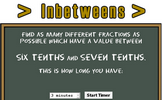#### Inbetweens

Find fractions between two given values.

### 7th Lesson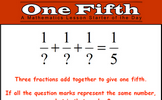#### One Fifth

Investigate three fractions which add together to give one fifth.

### 8th Lesson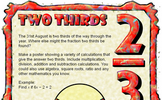#### Two Thirds

Make a poster showing a variety of calculations that give the answer two thirds.

### 9th Lesson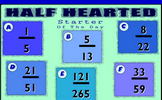#### Half Hearted

Find the number which when added to the top (numerator) and bottom (denominator) of each fraction make it equivalent to one half.

Some of the Starters above are to reinforce concepts learnt, others are to introduce new ideas while others are on unrelated topics designed for retrieval practice or and opportunity to develop problem-solving skills.

White Rose ResourcesEnd of block assessments provide a quick progress check at the end of each block of learning to make sure students have understood the content covered. This Scheme of Learning was produced by White Rose Maths and is used here with permission granted on 30th June 2021.For All: